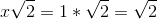## Example Questions

### Example Question #6 : How To Find The Length Of The Diagonal Of A Square

The perimeter of a square is 48. What is the length of its diagonal?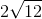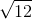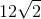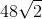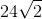Explanation:

Perimeter = side * 4

48 = side * 4

Side = 12

We can break up the square into two equal right triangles. The diagonal of the sqaure is then the hypotenuse of these two triangles.

Therefore, we can use the Pythagorean Theorem to solve for the diagonal: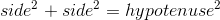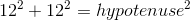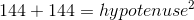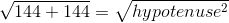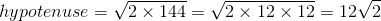### Example Question #1 : How To Find The Length Of The Diagonal Of A Square

What is the area of a square with a diagonal of length? Round to the nearest hundredth.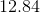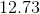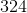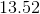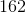Explanation:

Based on the data provided, the square could be drawn like this: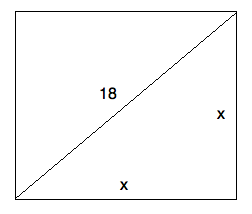Based on this, you can use the Pythagorean theorem to find: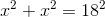or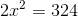From this, you know that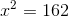Since the area is equal to, this is your answer!

### Example Question #7 : How To Find The Length Of The Diagonal Of A Square

The perimeter of a square isunits. How many units long is the diagonal of the square?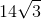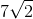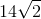Explanation:

From the perimeter, we can find the length of each side of the square. The side lengths of a square are equal by definition therefore, the perimeter can be rewritten as,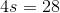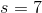Then we use the Pythagorean Theorme to find the diagonal, which is the hypotenuse of a right triangle with each leg being a side of the square.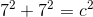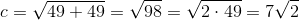### Example Question #1 : How To Find The Length Of The Diagonal Of A Square

Find the diagonal of a square with side length.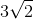Explanation:

To solve, simply realize the triangle that is made bysides and the diagonal is an isoceles right triangle. Thus, the hypotenuse is side length time square root of. Thus,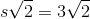### Example Question #2 : How To Find The Length Of The Diagonal Of A Square

Find the length of a diagonal of a square whose side length is.